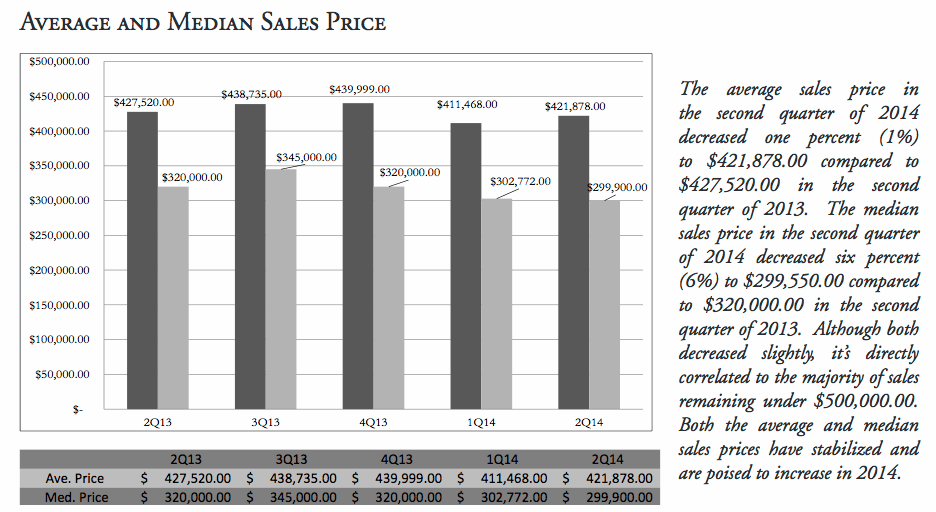# Median vs. Average

What is the difference between median vs. average values? How does this affect your pricing strategy?The median of a set of numbers is that number where half the numbers are lower and half the numbers are higher. In the case of real estate, that means that the median is the price where half the homes sold that month were cheaper, and half were more expensive. The average of a set of numbers is the total of those numbers divided by the number of items in that set. The median and the average might be close and they might not. It all depends on the numbers.

## Example:

Here are 11 fictional listing home prices.

1. \$100,000
2. \$101,000
3. \$102,000
4. \$103,000
5. \$104,000
6. \$105,000 ……………………….Median (middle)
7. \$106,000
8. \$107,000
9. \$650,000
10. \$1,000,000
11. \$3,000,000

The average price of these 11 homes added up & divided by 11 = \$498,000 is the Average Price of the 11 homes. (mean)

The median is quite easy to understand and calculate.  Look at our list of active listing prices. We need to arrange this list in order from lowest price to highest. Once that is done, simply find the value that’s in the exact middle of the list. That value is our median asking price! The median price of these 11 homes is \$105,000. Five homes were lower priced and five homes were higher priced.

The “average”, also known as the “mean”. This statistic is fairly easy to understand, as well as, to calculate. The average price of these 11 homes is \$498,000. That’s what you get if you add up all those prices and divide by 11.

What a difference! When you are looking at home prices, make sure you know whether the numbers are averages or medians. Both numbers provide good information, but they have different implications.